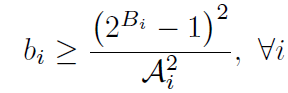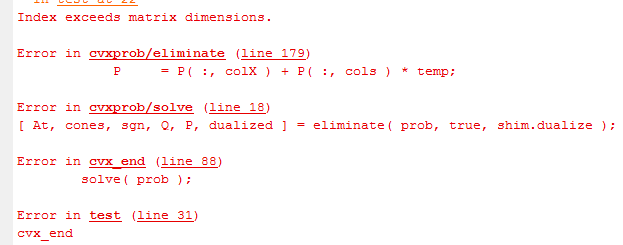# How to write my power constraint in CVX?

How to write this constraint in CVXActually, b, B, A are vectors of dimension M.

I try to write like this:
for i = 1:M
% b(i,1) >= (2^B(i)-1)^2/Alength(i)^2;
[1, t(i); t(i), b(i,1)*Alength(i,1)^2] >= 0
t(i) >= 2^B(i,1)-1
end
But CVX gives me the error message asWho can tell me why? Thanks in advance.

A post was merged into an existing topic: CVX_SDP Index exceeds matrix dimensions problem